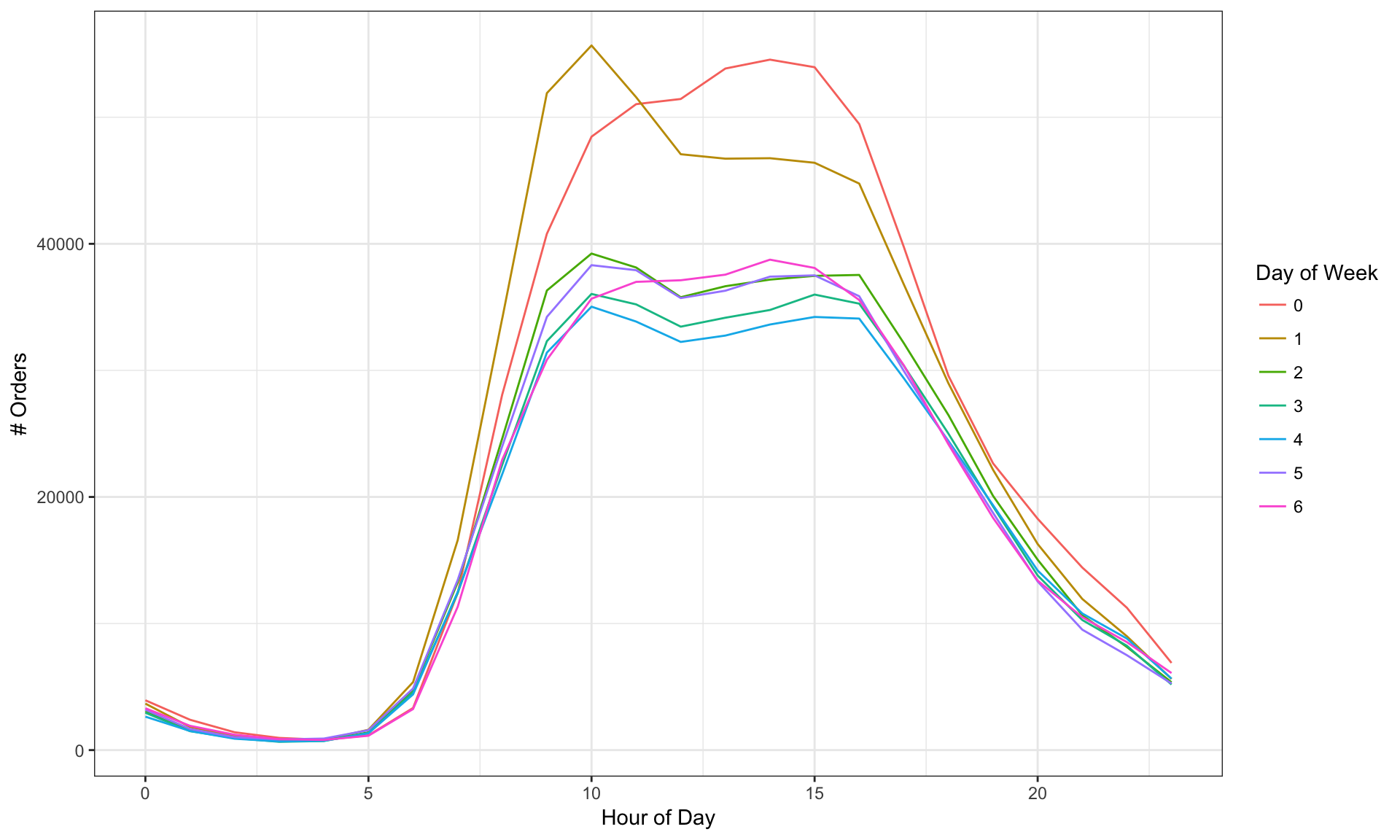Recently, Instacart released a dataset of ~3 million orders made by ~200,000 users at different days of week and times of day. There is also an ongoing Kaggle competition to predict which products a user will buy again. My goal here is more modest where I just wanted to explore the dataset to find patterns of purchasing behaviour by hour of day, day of week and number of days since prior order. An example of this kind of analysis is also shown in their blog. Here I wanted to explore if I can find such kind of patters by using the very common and popular dimension reduction technique - Principal Component Analysis (PCA). There are several great resources that introduce PCA if you are not familiar with PCA. One of the resources is the set of video lectures on machine learning by Prof. Hastie and Prof. Tibshirani.

The general approach that I have followed is:

• Do principal component analysis on the data (each row is a product, each column is a time period (hour of day, day of week or number of days since prior order))
• Review the loading plots of first two principal components to see purchase patterns and check the variance accounted by them.
• Identify top 20 products that have high absolute scores in either first or the second principal component
• Check the purchasing pattern by computing the average number of orders in each time period for the products that were identified as having top scores in one of the principal components.

Spoiler Alert: Since my analysis is basic, don’t be disappointed if there are no big Aha moments (there will be none). But I think it is still fun to see how we can extract such information directly from data.

We first load the libraries needed for the analysis

``````# load libraries
library(dplyr)
library(ggplot2)
library(scales)
library(tidyr)
library(htmlTable)

# source functions that are used for PCA analysis, extracting key information from PCA results
source("pca_fns.R")``````

I downloaded the data from the following link. The data dictionary is in the following link. Since the datasets are big, they are stored in tmpdata folder and not currently in the git repository. So somebody trying to reproduce this code will need to manually download the data and extract it to tmpdata subdirectory to be able to run this code.

``````# load datasets

orders\$order_hour_of_day = as.numeric(orders\$order_hour_of_day)

# stack order-product datasets (prior and train) together
ordprd = bind_rows(ordprd_prior, ordprd_train)

# Basic Data Info
#

# number of users
length(unique(orders\$user_id))``````
``##  206209``
``````# number of orders
nrow(orders)``````
``##  3421083``

Below are some basic info on the datasets

• The number of users are ~200,000.
• The number of orders are ~3.4M.

Next, we find products that account for top 80% of orders. We will work with this product set through the rest of the analysis

``````# Find products that account for top 80% of total orders
prod_orders = ordprd %>% group_by(product_id) %>% summarize(numorders = n())
prod_orders = inner_join(prod_orders, prods, by = "product_id")
prod_orders = prod_orders %>% arrange(desc(numorders)) %>% mutate(pctorders = cumsum(numorders)/sum(numorders)*100)
prod_top80pct = prod_orders %>% filter(prod_orders\$pctorders <= 80)``````

The number of products are ~50K or which ~5K account for 80% of total orders

## PCA to find patterns of purchase by hour of day

We first explore the number of orders by day of week and hour of day.

``````# orders by day of week/hour of day
wkhr_orders = orders %>% group_by(order_dow, order_hour_of_day) %>% summarize(numorders = n())

# Plot of orders by hour of day for each week of day
ggplot(data = wkhr_orders) +
geom_line(aes(x = order_hour_of_day, y = numorders, color = factor(order_dow))) +
xlab("Hour of Day") + ylab("# Orders") + scale_color_discrete(name = "Day of Week") +
theme_bw() ``````It is not clear whether day 0 is Saturday or Sunday. Since the number of orders is higher for days 0 and 1, maybe 0 and 1 are Saturday and Sunday. But certain other analysis further down seem to indicate that 0 is Sunday.

``````# create a dataset that calculates for each product, the percentage of product orders at each hour of day
ordprd2 = inner_join(ordprd, orders[,c("order_id","order_hour_of_day")], by = "order_id")
ordprd2a = ordprd2 %>% group_by(product_id, order_hour_of_day) %>%
summarize(numorders = n()) %>% mutate(pctorders = numorders/sum(numorders))
ordprd2a = inner_join(ordprd2a, prod_top80pct[,c("product_id"), drop = FALSE], by = "product_id")``````

Find products with different patterns of purchase timing by hour of day with PCA. Dataset for PCA has for each product (rows), the percentage of product orders at each hour of day (column)

``ordprd2a_s = ordprd2a %>% select(-numorders) %>% spread(order_hour_of_day, pctorders, fill = 0)``

Here I am plotting the average purchasing pattern by hour of day for a sample product item (chocolate sandwich cookies).

``````# Plot a row (product_id - 1, chocolate sandwich cookies)
ordprd2a_sample = data.frame(hour_of_day = names(ordprd2a_s)[-1], pctorders = t(ordprd2a_s[ordprd2a_s\$product_id == 24852,-1]))
ggplot() + geom_line(data = ordprd2a_sample, aes(x = as.numeric(hour_of_day), y = pctorders)) + theme_bw()``````